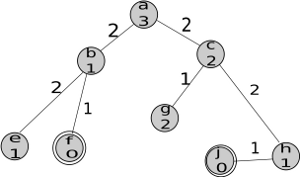Practice Exercise 3.E
Branch and Bound Search

## 2: Learning Goals

• Implement pruning

## 3: Directed Questions

1. In branch and bound (B&B), how is the upper bound (UB) calculated? [solution]

2. How is the lower bound (LB) calculated for a path? [solution]

3. With B&B, when do we prune a path? [solution]

## 4: Exercise: Branch and Bound Search

Consider the search problem represented in the following figure, where a is the start node and there are goal nodes at f and j. For each node, the heuristic cost is indicated on the node, and for each arc, the arc cost is indicated along the arc. Neighbors are ordered according to the f function.1. What is the UB when only the start node has been explored? [solution]

2. Which goal node is found first by B&B? [solution]

3. What is the UB immediately after the first goal node is found? [solution]

4. Is the second goal found by B&B? [solution]